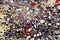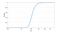Photo by Benny Jackson on Unsplash

# Can you identify me from a crowd?

## A modern “Where’s Waldo?” problem

When everyone’s private lives’ are on stored on Google’s servers and displayed on Facebook, the question arises “Could someone identify me from the crowd?” In a different example, at the check-out counter, when the cashier asks for your postal (zip) code, he asks information to be able to categorize you. Is that enough information to identify you? Clearly it depends on how much data you have shared intentionally or otherwise. So the real question is

How much data do you need to identify a single person?

Let us try to resolve the problem by careful analysis. Be prepared, there is going to be some math involved; I assume that readers are familiar with basics of linear algebra, information theory and statistics. If you are not brave enough for the adventure, then you’re welcome to skip to the end where I’ll highlight the main points.

## A sample of your data

So someone is trying to find you. For that purpose, he would need some information about you. To analyse this problem, we need some way of characterizing that information. Say that a vector x of length N contains all information about you in a binary form represented as +1's and -1's. With N bits we can uniquely describe 2^N individuals. Suppose further that we have a population of S individuals, each described by a similar vector x_k, where k is the index of the individual. We must then assume that log2(S)<N, such that every individual has a unique identifier x_k.

That someone has then access to a small subset of the data. He sees a length K vector y=Ax, where A is an unknown matrix of size KxN. The observation is much smaller than the entire data, K<N. Since we do not know anything about A, we can just as well assume that it is a random matrix chosen such that y is binary as well. It follows that the rows of A are approximately orthogonal. The covariance of y is then approximately equal to identity

The point is that a random subset of the data can be assumed to be uncorrelated with itself. Conversely, if we treat y_k as the binary representation of an integer number, then every integer in the range 0 to (2^K)-1 have equal probability. Naturally if the observation size is smaller than the population, K<log2(S), then we have no hope of identifying an individual. But the real question is, what is the likelihood that an individual is identifiable if we have a larger amount of data K>log2(S)?

## Does this sample uniquely identify you?

The real question then is that, given an integer y_1, what is the likelihood that none of the integers y_2 to y_P are equal to y_1, assuming that integers are uniformly distributed on 0 to (2^K)-1? Since y_1 is one out of 2^K, then clearly the probability that y_2 is not equal to y_1 is

P[one]=((2^K)-1)/(2^K) = 1-(2^-K)

Similarly, the probability that none of the integers y_2 to y_P are equal to y_1 is

P[all] = P[one]^(S-1) = (1-(2^-K))^(S-1).

To get a feel for what this means, let us make an experiment with a population of one million people, S=10⁶.Probability P[all] that you are identifiable in a population of S=10⁶ with K bits of information.

When we have K>20 bits of information, than the likelihood that you are identifiable is over 50%. With K>26 bits that likelihood is over 99%. Similarly, 99.9% and 99.99% are achieved with K>30 and K>33 respectively.

With the 99.9% threshold, we can then give a rule of thumb;

In a population of S individuals, you become identifiable once there is at least K=1.5 log2(S) bits of information.

## How realistic is this?

Given that Google and Facebook can easily have gigabytes of information about you, how realistic is the above analysis? First of all, note that Google and Facebook already can identify you. You’ve given your name (even if it would be a fake name) when you signed up. You have thus established an online identity. This is therefore the wrong place to look at.

The interesting question is whether you can be identified when you are not logged in. We’re back at the cashier who asks for your zip code. You didn’t tell your name to the cashier, so you’d be inclined to believe that you are anonymous. How much can it hurt to reveal your zip code? Depending on your country, zip codes have up to 10 digits, representing K=33 bits of information. You would then be identifiable in a population of roughly 4.2 million people.

The question however remains; is this realistic? Short answer: No. At the cashier, most people are from the surrounding area where zip codes are similar. Many people will have the same zip code. In practice, the shop would have plenty of other information about such that you would still be identifiable, but that is a different question. The point is that we assumed that our information is uniformly distributed, whereas zip codes are not.

For example, suppose the shop is visited by people from say an area covering 100 different zip codes, representing 7 bits of information. You’d be then identifiable in a population of S>25. Not too bad. So it really comes down to how much other information the shop has about you.

## Back to Maths — a Compression Problem

The difficult seems to be that even if we have a given amount of information, like a zip code of 33 bits, it is not clear how much unique information that contains. Our model was y=Ax, where we assumed that A is a random matrix, which in practice makes it likely that it is full-rank. To better correspond with reality, we should modify the problem to z=By=BAx, where z is the data which we observe and y is the subset of data from which the observation is drawn. Here z can be much larger than y.

We can think of y as a compressed version of the observation z. It contains all the information of the observation in the least number of bits. To determine how much information z reveals about you, we would then have to find the best possible compression. Once z is compressed into y, we can count the number of bits and we can again use the above rule of thumb to determine whether you are identifiable.

With compression, I here refer to packing the message as tightly as possible. You are probably familiar with the zip file format, available with tools like gzip and 7z. They are lossless tools, where the original file can be recovered exactly from the compressed file. Another type of compression is lossy coding like in MP3 files, where the recovered audio sounds similar to the original.

How much you can compress data is then very much dependent on the type of data. Text data can usually be compressed to about 15% of its original size (85% compression rate), while lossy audio codecs can be chosen to give a compression rate as low as 2% (though that usually gives a clearly audible reduction in quality).

## Statistical Modelling and Probability Distributions

Another issue is that above, we described binary data where every person has a black and white identifier. Real-life data is often not like that. Say we have data about your shopping habits. From the grocery store you often buy milk, cereals, bread and a few tomatoes. But not always. Sometimes you buy beer, a beauty magazine and candles. Most people are like that, they buy different items at different times.

Rather than bits, we should therefore discuss probability distributions; like what is the probability that you buy a beer from the shop? It does not really matter whether you actually bought beer or not, that event does not identify you, but we should evaluate whether it is likely that you are the person who bought this combination of items. This is a much more refined (=complicated) question.

Where above, we defined identifiability as the question of whether an observation can be linked to you with some probability threshold, here we consider whether an observation of you fits the a model which describes you. In other words, the new thing here is that we need a statistical model of your behaviour.

In theory we then would need a statistical model for every member of the population. Let’s say that we now get an observation, like the list of all items you bought from the shop. For each member of the population, we would then evaluate the probability of this shopping list. If there is a single individual for whom this shopping list fits much better than for the others, than we have identified our individual.

To build such statistical models, we clearly need lots of data about you. That is what Google and Facebook are doing. They hoard in as much data as they can. All data they find makes their statistical models more accurate. With more accurate models they can more easily identify you.

## Conclusions

I wanted to find out how much data one would need about me to be able to identify me in a crowd. It is like the classic “Where’s Wally?” problem. The outcome is a rule of thumb that the needed number of bits is 1.5 times the number of bits required to encode the population size. However, there are two drawbacks of this analysis. Firstly, you really should consider the maximally compressed data instead of plain data, though compression efficiency is very dependent on the data type. Secondly, typical data is probabilistic in nature — like the contents of your shopping list, it is not always the same — and the above rule of thumb fits poorly to such data. The better formulation of the question would then be:

Suppose a service has collected a database of background data for its users. The service then makes an observation of user activity. How much background and observation data does it need to identify that user in a given population?

My main conclusion is thus that identifiability is not only a function of observation and population size, but also very much a function of the size of background information database.

Written by

## Tom Bäckström

#### An excited researcher of life and everything. Professor of Practice in Speech Processing at Aalto University, Finland.

Welcome to a place where words matter. On Medium, smart voices and original ideas take center stage - with no ads in sight. Watch
Follow all the topics you care about, and we’ll deliver the best stories for you to your homepage and inbox. Explore
Get unlimited access to the best stories on Medium — and support writers while you’re at it. Just \$5/month. Upgrade